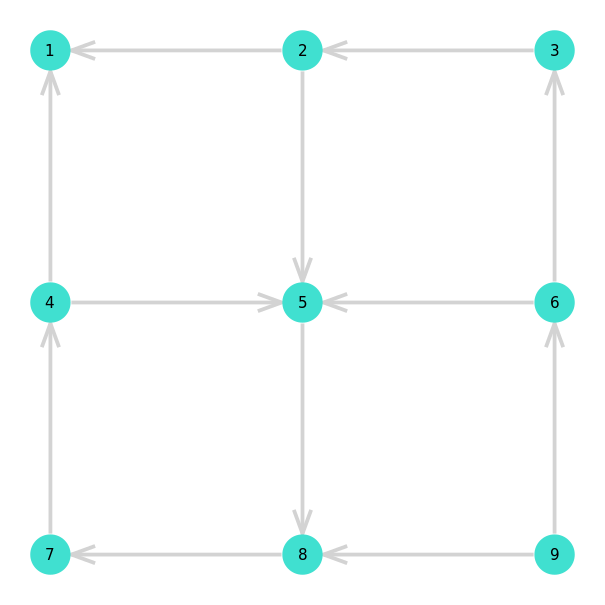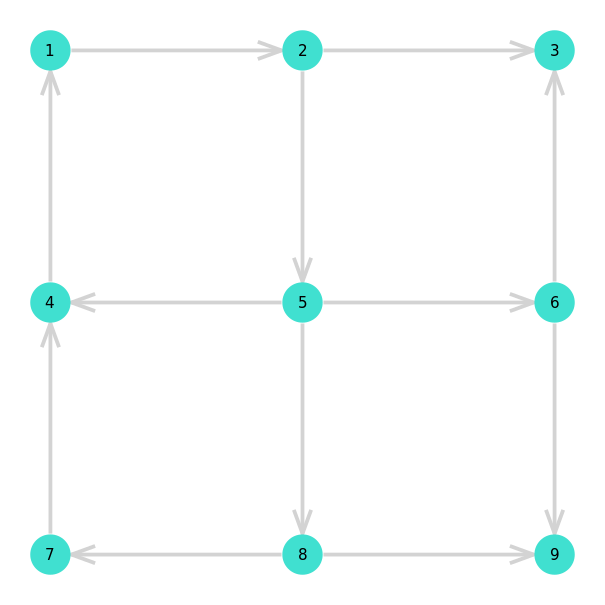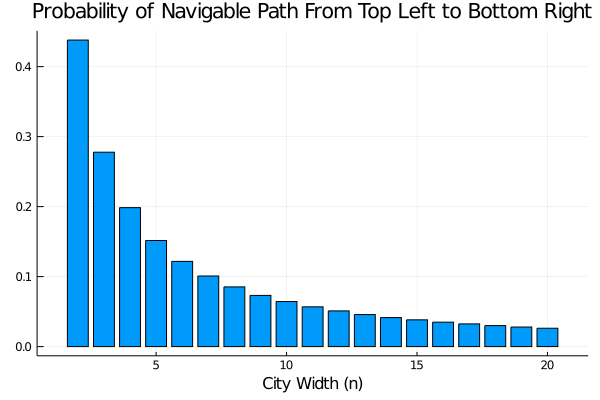In this post, I describe my (lazy) solution to FiveThirtyEight’s most recent Riddler Problem. This problem asks us to imagine living in a city which has a square gridded network of one-way streets whose orientations were assigned completely at random. Supposing that you live in the upper left corner of the city and work in the lower right corner, we’re asked: How likely is it that you can navigate from your home to work via the city’s street network?

The original prompt asked us to consider a square city two blocks across by two blocks high, however I chose to approach the problem more generally, considering square cities with an arbitrary width. As usual for these sorts of problems, I approached the problem from two directions, coming up with an exact analytical solution, and then estimating the result by simulation.

Unfortunately, I couldn’t think of an elegant exact solution… so I resorted to a brute force approach, simply enumerating all possible city layouts and counting the fraction with a navigable path from home to work. The brute force nature of my exact approach meant that I could only consider relatively small cities. In fact, letting $$n$$ denote the number of intersections across the city (i.e. one more than the number of city blocks!), I was only able to “solve” the problem for $$n = 2,3,4$$. But, because this range included the $$n = 3$$ case discussed in the prompt, I decided to call it quits for exact methods after that 🙂

More interestingly, for cities of size $$n = 2, \ldots, 20$$, I estimated the probability that you could navigate by simulating random street assignments and counting the fraction that had a navigable path from home to work. In principle, this approach would have worked for any city size, but I figured $$20$$ was big enough.

As usual, I’ve made the script for my solution available, and you download the solution script (in Julia) here. This time, I wrote my solution as a Pluto notebook, which I’ve rendered statically for viewing here.

## Simulation Solution

To begin with, lets take a look at a couple realizations of random street assignments for the $$2 \times 2$$ block / $$n = 3$$ case. In the first example, there is no path from home (labeled $$1$$) to work (labeled $$9$$). In the second example, you can get to work using the path 1 => 2 => 5 => 8 => 9 or 1 => 2 => 5 => 6 => 9. Unfortunately, although you can get to work in this city, there isn’t any way for you to get back home!For each random street assignment, I determined whether there was a path from home to work by constructing a directed graph from the street assignment using LightGraphs.jl. From there, I determined whether the network had a path from home to work using the very aptly named has_path function.

For each city size $$n = 2, 3, \ldots, 20$$, I generated one million random street assignments and determined the fraction which had a navigable path from home to work. The results are summarized in the following figure.The following table summarizes the results for small $$n$$, and the complete data set can be downloaded here.

 City Width Probability Navigable 2 0.437949 3 0.277747 4 0.198520

So, we see that the solution for $$n = 3$$ is around $$27.7\%$$.

## (Lazy) Exact Solution for Small Cities

As I mentioned in the introduction, I couldn’t think of a good analytical solution to this problem, so I decided to implement a bad solution - i.e. I just brute forced the answer. If the problem had asked for a slightly larger city, say a $$4 \times 4$$ grid (i.e. $$n = 5$$) then this approach would not have been feasible. However, for cities of size $$n = 2,3,4$$ there are only $$2^4, 2^{12}$$, and $$2^{24} = 16,777,216$$ street layouts respectively1, so these can all be easily brute-forced.

To perform the computation, I followed largely the same steps that I discussed in the previous section. The only difference here, was that I looped over all possible street layouts instead of generating them at random.

The following table shows the results:

 City Size Probability Navigable 2 $$7 / 16 \approx 0.4375$$ 3 $$1,135 / 4,096 \approx 0.2771$$ 4 $$3,329,245 / 16,777,216 \approx 0.1984$$

From the table, we see that for a $$2\times2$$ city (i.e. $$n = 3$$) there’s about a $$27.7\%$$ chance that we’ll be able to drive to work!

## Footnotes

1. To see this, first draw out the cities and convince yourself that cities of these sizes respectively have a total of $$4, 12$$ and $$24$$ streets. From there, note that, because each street can be in one of two directions there are $$2^{n_\text{streets}}$$ possible layouts for any city.